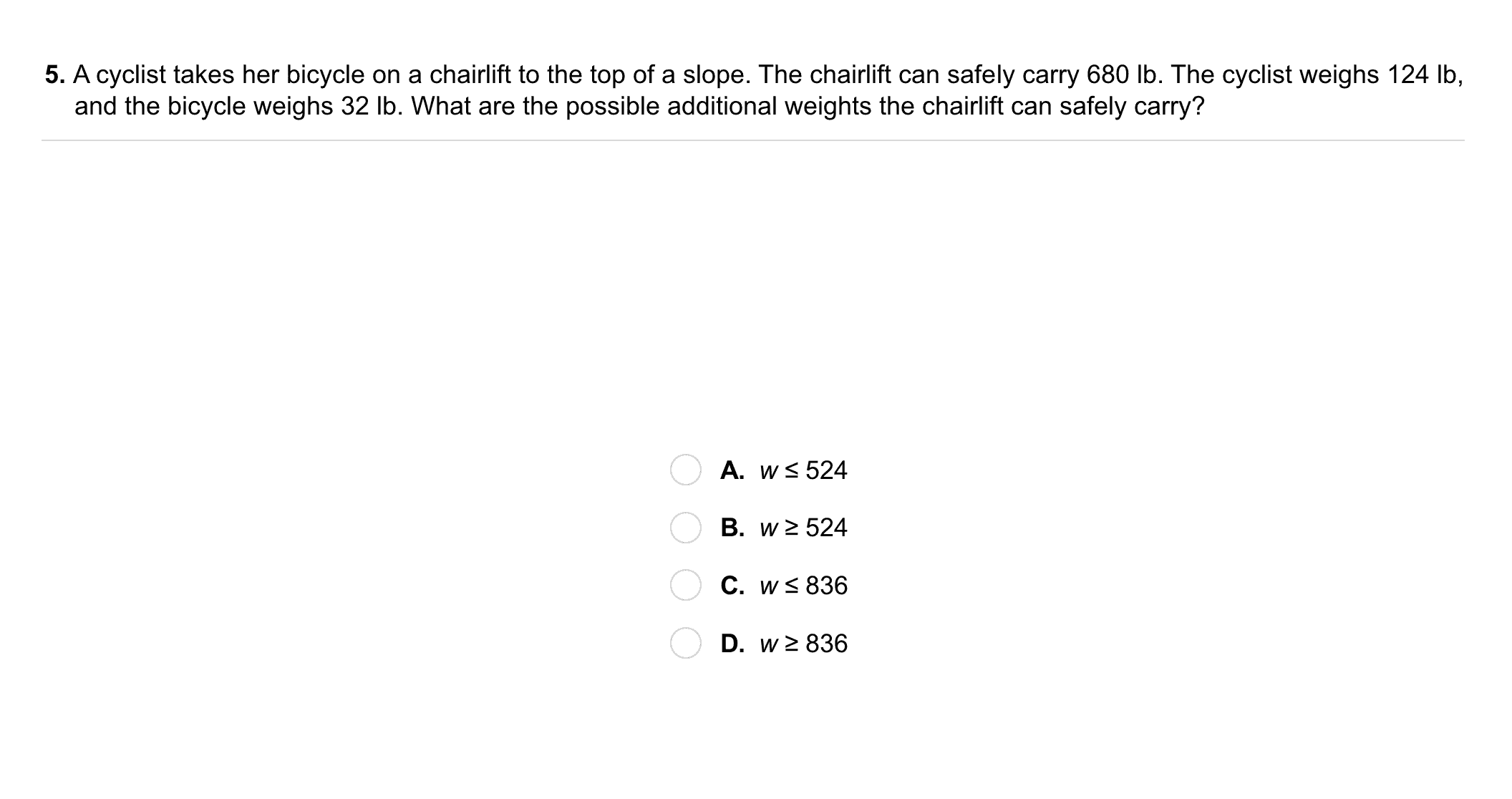Algebra 1 3-2 Complete Lesson: Solving Inequalities Using Addition or Subtraction
starstarstarstarstarstarstarstarstarstar
3 (2 ratings)
by Matthew Richardson
| 27 Questions
Note from the author:
A complete formative lesson with embedded slideshow, mini lecture screencasts, checks for understanding, practice items, mixed review, and reflection. I create these assignments to supplement each lesson of Pearson's Common Core Edition Algebra 1, Algebra 2, and Geometry courses. See also mathquest.net and twitter.com/mathquestEDU.The outlined content above was added from outside of Formative.1
1
10 pts
Solve It! What is the least number of states in the southeastern U.S. that the candidate could win and still become president?
Enter only a number.
1
2
3
4
52
10 pts
Problem 1 Got It? Solve the inequality, showing your step(s), and graph the solutions on the number line.
Be sure to include all relevant graph detail.
3
10 pts
Problem 2 Got It? Solve the inequality, showing your step(s), and graph the solutions on the number line. Check your solutions.
Be sure to include all relevant graph detail.4
10 pts
Problem 3 Got It? Solve the inequality, showing your step(s), and graph the solutions on the number line. Check your solutions.
Be sure to include all relevant graph detail.5
5
10 pts
Problem 4 Got It?
A
B
C
D6
6
10 pts
Problem 4 Got It?
A
B
C
D7
10 pts
Solve the inequality. Then graph and check your solution.
Include all relevant graph detail.
8
10 pts
Solve the inequality. Then graph and check your solution.
Include all relevant graph detail.
9
10 pts
Solve the inequality. Then graph and check your solution.
Include all relevant graph detail.
10
10 pts
Solve the inequality. Then graph and check your solution.
Include all relevant graph detail.11
11
10 pts
A
B
C
D12
10 pts
Writing: How can you use the addition and subtraction properties of inequality to produce equivalent inequalities?
13
30 pts
Reasoning: What can you do to the first inequality in each pair in order to get the second inequality?

14
10 pts
Compare and Contrast: Suppose you solve the two inequalities:

How are your methods of solving the inequalities similar? How are they different?15
10 pts
Review Lesson 3-1: Define a variable and write an inequality to model the situation.

A hummingbird migrates more than 1850 mi.
Let d represent the distance in miles that a hummingbird migrates; d > 1850
Let m represent the distance in miles that a hummingbird migrates; m ≥ 1850
Let h represent the distance in miles that a hummingbird migrates; h < 1850
16
10 pts
Review Lesson 3-1: Define a variable and write an inequality to model the situation.

An octopus can be up to 18 ft long.
17
5 pts
Review Lesson 1-2: Simplify the expression.
Enter only a number.
18
5 pts
Review Lesson 1-2: Simplify the expression.
Enter only a number.
19
5 pts
Review Lesson 1-2: Simplify the expression.
Enter only a number, in decimal form.
20
5 pts
Review Lesson 2-1: Solve the equation.
Enter only a number.
21
5 pts
Review Lesson 2-1: Solve the equation.
Enter only a number, in this form: -21/4
22
10 pts
Review Lesson 2-1: Solve the equation.
Enter only a number in simplest form.23
10 pts
Vocabulary Review: Match each verbal description on the left with its inequality symbol on the right.
• less than
• at most
• no greater than
• fewer than
• more than
• no more than
• greater than
• as little as
• greater than or equal to
• at least
• less than or equal to
• no less than
• <
• >
• <
• >24
10 pts
Use Your Vocabulary: Categorize each of the equations.
• 3 = x
• x = 1/3
• x + 2 = 5
• x + 2 = 5 - 2
• Equivalent to the equation x = 3
• Not equivalent to the equation x = 3
25
10 pts
Use Your Vocabulary: Categorize each of the inequalities.
• 3 ≥ x
• x ≤ 1/3
• x + 2 ≥ 5
• x + 2 ≤ 5 - 2
• Equivalent to x ≤ 3
• Not equivalent to x ≤ 3
26
100 pts
Notes: Take a clear picture or screenshot of your Cornell notes for this lesson. Upload it to the canvas. Zoom and pan as needed.

For a refresher on the Cornell note-taking system, click here.
27
10 pts
Reflection: Math Success
Add to my formatives list

Formative uses cookies to allow us to better understand how the site is used. By continuing to use this site, you consent to the Terms of Service and Privacy Policy.